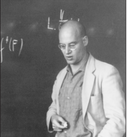Select Page

# An introduction to derivators with applications to the study of t-structures

Dates

2 – 6 May 2019
Thu 2 May: 11.00-13.00h
Fri 3 May: 11.00-13.00h
Mon 26 May: 11.00-13.00h

Location

Aula 1
Centre de Recerca Matemàtica
(CRM)

Abstract

The theory of stable derivators can be thought of as a minimal enhancement of triangulated categories that allows for a good theory of homotopy co/limits. The idea of a derivator has been introduced independently by several authors in different areas of Pure Mathematics:  Heller (in Algebraic Topology), Grothendieck (in Algebraic Geometry), Franke, Keller (in Representation Theory), and possibly others. This theory has recently found several applications to classical problems that had remained open for a long
time in the setting of triangulated categories.

In the first class of this short course will first recall the needed background in (2-)category theory and use it to introduce the notion of prederivator. We will then go on defining derivators, pointed derivators and strong derivators. In the context of pointed derivators we will present some basic constructions, such as the cone/fiber and the suspension/loop adjunctions. We will illustrate
the theory with some canonical examples.

The second class will be devoted to the concept of stability. We will start recalling some basic facts about triangulated categories, we will then introduce the notion of stability for an abstract pointed derivator and we will show that, when it is also strong, the images of a stable derivator are canonically triangulated. In this way, a strong and stable derivator becomes an enhancement of a triangulated category, called its underlying category. We will illustrate the theory with a brief analysis of the natural derivator enhancing the
derived category of an Abelian category.

In the third and last class we will concentrate on a recent application of the theory of stable derivators. We will start recalling the notion of a t-structure on a general triangulated category and we will show how to put this classical notion in the setting of derivators. The advantage of this enhancement is that we will gain a good control of co/limits in the heart of a t-structure and, in this way, we
can give very natural sufficient conditions for the heart of a t-structure to be a Grothendieck category.

## LecturersDates

2 – 6 May 2019
Thu 2 May: 11.00-13.00h
Fri 3 May: 11.00-13.00h
Mon 26 May: 11.00-13.00h

Location

Aula 1
Centre de Recerca Matemàtica
(CRM)Abstract

The theory of stable derivators can be thought of as a minimal enhancement of triangulated categories that allows for a good theory of homotopy co/limits. The idea of a derivator has been introduced independently by several authors in different areas of Pure Mathematics:  Heller (in Algebraic Topology), Grothendieck (in Algebraic Geometry), Franke, Keller (in Representation Theory), and possibly others. This theory has recently found several applications to classical problems that had remained open for a long
time in the setting of triangulated categories.

In the first class of this short course will first recall the needed background in (2-)category theory and use it to introduce the notion of prederivator. We will then go on defining derivators, pointed derivators and strong derivators. In the context of pointed derivators we will present some basic constructions, such as the cone/fiber and the suspension/loop adjunctions. We will illustrate
the theory with some canonical examples.

The second class will be devoted to the concept of stability. We will start recalling some basic facts about triangulated categories, we will then introduce the notion of stability for an abstract pointed derivator and we will show that, when it is also strong, the images of a stable derivator are canonically triangulated. In this way, a strong and stable derivator becomes an enhancement of a triangulated category, called its underlying category. We will illustrate the theory with a brief analysis of the natural derivator enhancing the
derived category of an Abelian category.

In the third and last class we will concentrate on a recent application of the theory of stable derivators. We will start recalling the notion of a t-structure on a general triangulated category and we will show how to put this classical notion in the setting of derivators. The advantage of this enhancement is that we will gain a good control of co/limits in the heart of a t-structure and, in this way, we
can give very natural sufficient conditions for the heart of a t-structure to be a Grothendieck category.